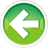- Learn C++ Test Site - https://test.learncpp.com -

# 8.1 — Introduction to compound data types

In lesson 4.1 -- Introduction to fundamental data types , we introduced fundamental data types, which are the basic data types that C++ provides as part of the core language.

We’ve made much use of these fundamental types in our programs so far, especially the `int` data type. And while these fundamental types are extremely useful for straightforward uses, they don’t cover our full range of needs as we begin to do more complicated things.

For example, imagine you were writing a math program to multiply two fractions. How would you represent a fraction in your program? You might use a pair of integers (one for the numerator, one for the denominator), like this:

And a run of this program:

```Enter a fraction: 1/2
Enter a fraction: 3/4
The two fractions multiplied: 3/8
```

While this program works in this limited case, it introduces a couple of challenges for us to solve. First, each pair of integers is only loosely linked -- outside of comments and the context of how it’s used in the code, there’s little to suggest that each numerator and denominator are related somehow. Second, following the DRY (don’t repeat yourself) principle, we should create a function to handle the user inputting a fraction (along with some error handling). However, functions can only return a single value, so how would we return the numerator and denominator back to the caller?

Now imagine another case where you’re writing a program that needs to keep a list of employee IDs. How might you do so? You might try something like this:

But what if you had 100 employees? First, you’d need to type in 100 variable names. And what if you needed to print them all? Or pass them to a function? We’d be in for a lot of typing. This simply doesn’t scale.

Clearly fundamental types will only carry us so far.

Compound data types

Fortunately, C++ supports a second set of data types called `compound data types`. Compound data types (also sometimes called composite data types) are data types that can be constructed from fundamental data types (or other compound data types). Each compound data types has its own unique properties as well.

As we’ll show in upcoming lessons, we can use compound data types to solve all of the challenges we presented above.

C++ supports the following compound types:

• Functions
• Arrays
• Pointer types:
• Pointer to object
• Pointer to function
• Pointer to member types:
• Pointer to data member
• Pointer to member function
• Reference types:
• L-value references
• R-value references
• Enumerated types:
• Unscoped enumerations
• Scoped enumerations
• Class types:
• Structs and classes
• Unions

You’ve already been using one compound type (likely without knowing it), as functions are a compound type. For example, consider this function:

The type of this function is `void(int, double)`. Note that this type is composed of fundamental types. Of course, functions also have their own special behaviors as well (e.g. being callable).

The vast majority of the functionality in the C++ standard library is implemented using two compound types: functions and classes. As an example, our old buddies `std::cout` and `std::cin` are actually class-type objects. And `operator<<`, which we’ve been using to output values to the console, is actually a function!

In this chapter, we’ll cover some of the more straightforward compound types, including `l-value references`, `pointers`, `unscoped enumerations`, `scoped enumerations`, and C-style `structs`.

Then, in the next chapter, we’ll introduce class types and dig into some of the more useful `array` types. This includes `std::string` (introduced in lesson 4.12 -- An introduction to std::string ), which is actually a class type!

Here we go!8.2 -- Value categories (r-values and l-values)Index8.x+-+Chapter+8+summary+and+quiz